# Percentages + multiplication - math problems

#### Number of problems found: 60

• Percentages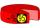Expressed as a percentage:
• Mixed with percentagesCalculate 33 1/3% of 570.
• Percentages above 100%What is 122% of 185? What is the meaning of percentages above 100%?
• Events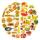Event P has probability of 0.84. What is the probability that the event P occurs in 3, 5, 7 try.
• Double percent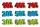What is 40% of 80% of 11800?
• travel agency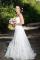Small travel agency offers 5 different tours at honeymoon. What is the probability that the bride and groom choose the same tour (they choose independently)?
• The ballThe ball was discounted by 10 percent and then again by 30 percent. How many percent of the original price is now?
• Rate or interestAt what rate percent will Rs.2000 amount to Rs.2315.25 in 3 years at compound interest?
• Associative law multiplicationIn a warehouse, you obtain a 20% discount but you must pay a 15% sales tax. Which would you prefer to have calculated first: discount or tax? Explain. (result write as: 1 = first discount, 2 = first tax, 3 = no matter what first)
• Percent calculationCalculate 51% if 99% is 79.
• Highway repairThe highway repair was planned for 15 days. However, it was reduced by 30%. How many days did the repair of the highway last?
• If weIf we increase the unknown number by 4%, we get 780. Determine the unknown number.
• Three colors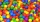Find the probability that 3 balls of the same color will be drawn from fate with 10 white, 10 red, and 10 blue balls.
• Discount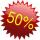Product has been discounted twice by 19%. What is the total discount given?
• Percent chaining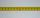Calculate 20% from 70% from 80 km
• Briefcase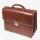The cost of producing briefcase is € 45. The manufacturer wants to sell it at a profit 30%. For how much will briefcase sell?
• Two acesFrom a 32 card box we randomly pick 1 card and then 2 more cards. What is the probability that last two drawn cards are aces?
• JUDr. Usury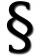Judge JUDr. Usury approved the agreement on guilt and punishment where confessed to pay daily interest 0.18%. How big is a yearly interest? Year has 360 days.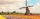How large must the group of people be so that the probability that two people have a birthday on the same day of the year is greater than 90%?
• Farmer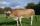The farmer farming 293 ha of arable land and the remaining 20% are meadows and pastures. How much land farmer owns?

Do you have an interesting mathematical word problem that you can't solve it? Submit a math problem, and we can try to solve it.

We will send a solution to your e-mail address. Solved examples are also published here. Please enter the e-mail correctly and check whether you don't have a full mailbox.

Please do not submit problems from current active competitions such as Mathematical Olympiad, correspondence seminars etc...